# HBSE 7th Class Maths Solutions Chapter 5 Lines and Angles InText Questions

Haryana State Board HBSE 7th Class Maths Solutions Chapter 5 Lines and Angles InText Questions and Answers.

## Haryana Board 7th Class Maths Solutions Chapter 5 Lines and Angles InText Questions

Try These (Page No. 94):

Question 1.
List ten figures around you and identify the acute, obtuse and right angles found in them.
Solution: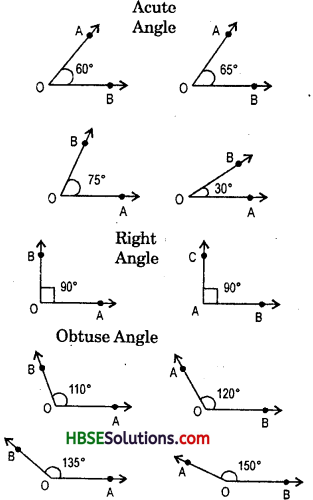Think, Discuss & Write (Page No. 95):

Question 1.
Can two acute angles be complement of each other ?
Solution:
Yes complementary 30° is and acute angle and 60° is also an acute angle.
30° + 60° = 90° (Complementary angle)

Question 2.
Can two obtuse angles be complement of each other ?
Solution:
No obtuse angle > 90°

Question 3.
Can two right angles be complement of each other ?
Solution:
No, one right angle = 90°

Try These (Page No. 95):

Question 1.
Which pairs of following angles are complementary ?
Solution:
(i) and (iv), because complementary angle = 90°.

Question 2.
What is the measure of the complement each of following given angles ?
(i) 45° (ii) 65° (iii) 41° (iv) 54°
Solution:
(i) Let the complement of 45° is re.
∴ x + 45° = 90°
or x = 90° -45° = 45°
∴ Complement of 45° is 45°.

(ii) Let the complement of 65° is x.
∴ x + 65° = 90°
or re = 90° -65° = 25°
∴ The complement of 65° is 25°.

(iii) Let the complement of 41° be m.
∴ m + 41° = 90°
or m = 90° – 41° = 49°
or m = 49°
Thus, the complement of 41° is 49°.(iv) Let the complement of 54° be x.
∴ x + 54° = 90°
or re = 90° – 54° = 36°
Thus, the complement of 54° is 36°.

Question 1.
The difference in the measures of two complementary angles is 12°. Find the measures of the angles.
Solution: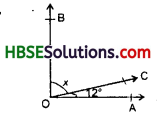90° -x = 12°
90° – 12° = x
or 78° = x
∴  x = 78°

Think, Discuss & Write (Page No. 96):

Question 1.
Can two obtuse angles be supplementary ?
Solution:
No, supplementary angle = 180°
obtuse > 90°.

Question 2.
Can two acute angles be supplementary ?
Solution:
Yes, acute angle < 90°

Question 3.
Can two right angles be supplementary ?
Solution:
Yes, right angle
= 90°

Try These (Page No. 97):

Question 1.
Find the supplementary angles in the following given pairs :
Solution:
(i) Measures of the given angles are 110° and 50°.
∵ 110°+ 50° = 160°.
and 160° ≠ 180°
∴ 110° and 50° are not a pair of supplementary angles.

(ii) Measures of the given angles are 105° and 65°.
∵ 105°+ 65° = 170°.
and 170° ≠ 180°
∴ 105° and 65° are not a pair of supplementary angles.

(iii) Measures of the given angles are 50° and 150°.
∵ 50° + 130° = 180°.
∴ 50° and 130° are a pair of supplementary angles.

(iv) Measures of the given angles are 45° and 45°.
∵ 45° + 45° = 90°. and 90° ≠ 180°
∴ 45° and 45° are not a pair of supplementary angles.Question 1.
What will be the measure of the supplement of each one of the following angles ?
(i) 100° (ii) 90° (iii) 55° (iv) 125°
Solution:
(i) Let the supplementary of 100° be a.
∴ 100° + x = 180°
or x = 180° – 100° = 80°
∴ The measure of the supplement of 100° is 80°.

(ii) Let the supplementary of 90° be x.
∴ x + 90° = 180°
or x = 180°- 90° = 90°
The measure of the supplement of 90° is 90°.

(iii) Let the supplementary of 55° be x.
∴ 55° + x = 180°
or x = 180° -55° = 125°
The supplement of 55° is 125°.

(iv) Let the supplementary of 125° bey.
∴ y + 125° = 180°
or y = 180°-125° = 55°
∴ The supplement of 125° is 55°.

Question 2.
Among two supplementary angles the measure of the larger angle is 44° more than the measure of the smaller. Find their measures.
Solution:
A-t-q, x + x + 44 = 180°
or, 2x + 44 = 180°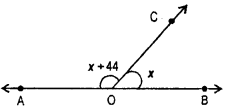2x = 180° – 44°
x = $$\frac{136}{2}$$ = 68°
∴ 68°, 112°

Try These (Page No. 97-98):

Question 1.
Are the angles marked 1 and 2 adjacent ? If they are not adjacent.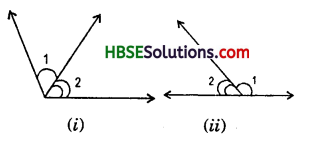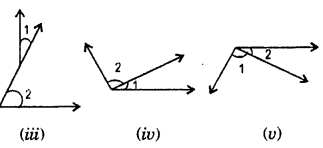Solution:
(i) Yes, (ii) Yes, (iii) No, (iv) Yes,
Yes.
(iii) No, because two angles in a plane are said to be adjacent angles, if they have a common vertex, a common arm and the other two arms on opposite sides of the common arm.Question 2.
In the given figure are the following adjacent angles ?
(a) ∠AOB and ∠BOC
(b) ∠BOD and ∠BOC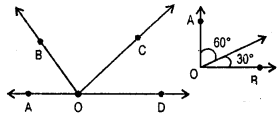Solution:
(a) Yes, because they have a common vertex O and a common arm OB.
(b) No, because they are not placed next to each other.

Think, Discuss & Write (Page No. 98):

Question 1.
Can two adjacent angles be supplementary ?
Solution:
Yes, because two adjacent angles form a supplementary.

Question 2.
Can two adjacent angles be complementary ?
Solution:
Y es, because two adjacent angles form a complementary.

Question 3.
Can two obtuse angles be adjacent angles ?
Solution:
Yes, in the figure, ∠BOC and ∠COD are obtuse angles and they are adjacent angles.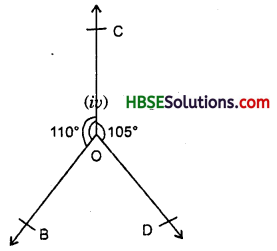Question 4.
Can an acute angle be adjacent to an obtuse angle ?
Solution:
Yes, in the figure, ∠1 and ∠2 are adjacent angles. ∠1 is acute and ∠2 is an obtuse angle.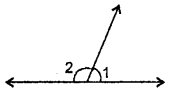Think, Discuss & Write (Page No. 99):

Question 1.
Can two acute angles form a linear pair ?
Solution:
No, because acute angle < 90° and linear pair = 180°. Question 2. Can two obtuse angles form a linear pair ? Solution: No, because obtuse angle > 90°
and linear pair = 180°.Question 2.
Can two right angles form a linear pair ?
Solution:
Yes, because right angle = 90°
and linear pair = 180°.

Try These (Page No. 99):

Question 1.
Check which of the following pairs of angles form a linear pair:
Solution:
(i) Yes,
∵ 140°+ 40° = 180°
∴ The given pair of angles form a linear pair.

(ii) No,
∵ 60° + 60° = 120°
and 120° ≠ 180°.

(iii) No,
∵ 90° + 80° = 170°
and 170° ≠ 180°.

(iv) Yes,
∵ 115°+ 65° = 180°.

Try These (Page No. 101):

Question 1.
In the given figure, if ∠1 = 30°. find ∠2 and ∠3.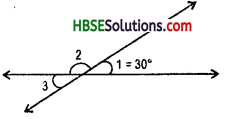Solution:
Since ∠1 + ∠2 = Linear pair = 180°
or, 30° + ∠2 = 180°
or, ∠2 = 180°-30°
∴ ∠2 = 150°
Now, ∠2 + ∠3 = 180°
or, 150° + ∠3 = 180°
or, ∠3 = 180° -150°
∴ ∠3 = 30°
or, ∠1 = ∠2 = vertically opposite angle
∴ 30° = ∠2
∴ ∠2 = 30°

Question 2.
Give an example for vertically opposite angles in your surroundings.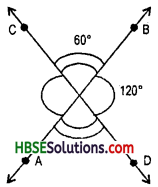Solution:
Two angles formed by two intersecting lines having common arm are said to be vertically opposite angles.
Hence, ∠COB = ∠AOD = 60°
= vertically opposite angle
and ∠BOD = ∠AOC = 120°
= vertically opposite anglesTry These (Page No. 104):

Question 1.
Find examples from your surround-ings where lines intersect at right angles.
Solution:
(i) A black board, (ii) A table, (iii) A television (iv) A computer.

Question 2.
Find the measures of the angles made by the intersecting lines at the vertices of an equilateral triangle.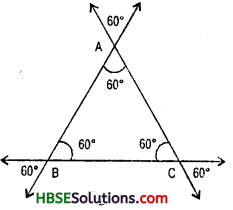Solution:
Vertices, A, B and C. (Fig.)

Question 3.
Draw any rectangle and find the measures of angles at the four vertices made by the intersecting lines.
Solution: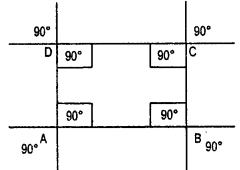Question 4.
If two lines intersect, do they always intersect at right angles ?
Solution: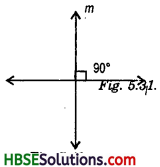Try These (Page No. 105):

Question 1.
Suppose Two lines are given. How many transversals can you draw for these lines.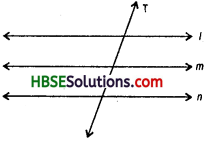Solution:
Many

Question 2.
If three lines have a transversal, how many points of intersections are there ?
Solution:
Three.Question 3.
Try to identify a few transversals in your surroundings.
Solution:

Try These (Page No. 1.6):

Question 1.
Name the pairs of angles in each Figure.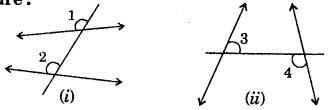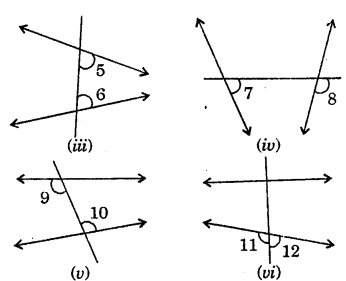Solution:
(ii) i.e. < 1 and < 2 are called pairs corresponding angles. [Fig. (i)]
(it) i.e. <3 and < 4 are called pairs of alternate interior angles. [Fig. (ii)]
(iii) i.e. < 5 and < 6 are called pairs of interior angles on the same side of the transversal. [Fig. (iii)]
(iv) i.e. < 7 and < 8 are called pairs of corresponding angles. [Fig.(iv)]
(v) i.e. < 9 and < 8 are called pairs of alternate interior angles. [Fig. (v)]
(vi) i.e. < 11 and < 12 are called linear pairs. [Fig. (vi)]

Try These (Page No. 109):

Question 1.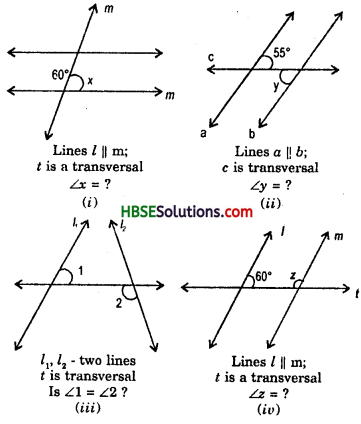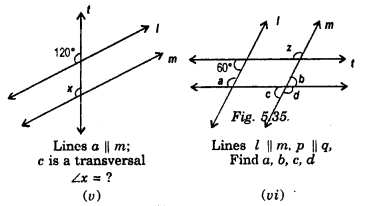Solution:
(i) x = 60°
[∵ x and 60° are alternate interior angles]

(ii) ∠y = 55°
[∵ y and 55° are alternate interior angles]

(iii) If two non-parallel lines are intersected by a transversal, then the angle of pairs of alternate interior angles are not equal.
Hence ∠1 = ∠2

(iv) 60° + z = 180°
⇒ z = 180° – 60°
z = 120°
x = 120°
[ ∵ and 60° are interior angles on the same side of the transversal]
∴ z = 120°.

(v) x = 120°
[∵ x and 120° are corresponding angles]

(vi) a + 60° = 180°
⇒ a = 180° – 60° = 120°
a – c
⇒ c = 120° [alternate angles]
c = b
[vertically opp. angles]
⇒ b = 120° [linear pair]
⇒ b+d = 180°
⇒ 120° + d = 180°
∴ d = 180° – 120° = 60°.Try These (Page No. 100):

Question 1.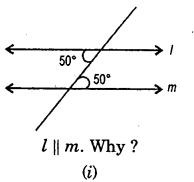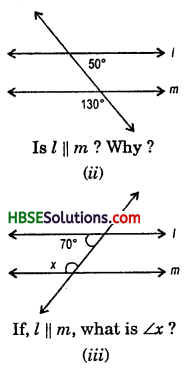Solution:
(i) Yes, l || m, because alternate interior angles are equal and 50°.
(ii) Yes, l || m, because alternate interior angles are equal and 50°.
(iii) If two parallel lines are intersected by a transversal, then the sum of the interior angles on the same side of the transversal is 180° or supplementary.
Hence, 180° + x = 180°
or, x = 180° -180°
x = 0°
But in this figure one angle is 180°. Hence it is not possible.